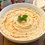# Hint: Zeta is involved!

$\large \displaystyle\sum _{ n=1 }^{ \infty }{ \sum _{ k=1 }^{ \infty }{ \dfrac { 1 }{ \left\lfloor \sqrt { n+k } \right\rfloor ^{ a } } } }$

Find a closed form for the above summation for $a>4$.

This is a part of the set Formidable Series and IntegralsNote by Hamza A
5 years, 2 months ago

This discussion board is a place to discuss our Daily Challenges and the math and science related to those challenges. Explanations are more than just a solution — they should explain the steps and thinking strategies that you used to obtain the solution. Comments should further the discussion of math and science.

When posting on Brilliant:

• Use the emojis to react to an explanation, whether you're congratulating a job well done , or just really confused .
• Ask specific questions about the challenge or the steps in somebody's explanation. Well-posed questions can add a lot to the discussion, but posting "I don't understand!" doesn't help anyone.
• Try to contribute something new to the discussion, whether it is an extension, generalization or other idea related to the challenge.
• Stay on topic — we're all here to learn more about math and science, not to hear about your favorite get-rich-quick scheme or current world events.

MarkdownAppears as
*italics* or _italics_ italics
**bold** or __bold__ bold
- bulleted- list
• bulleted
• list
1. numbered2. list
1. numbered
2. list
Note: you must add a full line of space before and after lists for them to show up correctly
paragraph 1paragraph 2

paragraph 1

paragraph 2

[example link](https://brilliant.org)example link
> This is a quote
This is a quote
    # I indented these lines
# 4 spaces, and now they show
# up as a code block.

print "hello world"
# I indented these lines
# 4 spaces, and now they show
# up as a code block.

print "hello world"
MathAppears as
Remember to wrap math in $$ ... $$ or $ ... $ to ensure proper formatting.
2 \times 3 $2 \times 3$
2^{34} $2^{34}$
a_{i-1} $a_{i-1}$
\frac{2}{3} $\frac{2}{3}$
\sqrt{2} $\sqrt{2}$
\sum_{i=1}^3 $\sum_{i=1}^3$
\sin \theta $\sin \theta$
\boxed{123} $\boxed{123}$

## Comments

Sort by:

Top Newest

we have $\displaystyle\sum _{ n=1 }^{ \infty }{ \sum _{ k=1 }^{ \infty }{ \dfrac { 1 }{ \left\lfloor \sqrt { n+k } \right\rfloor ^{ a } } } } =\displaystyle\sum _{ i=1 }^{ \infty }{ \left( \displaystyle\sum _{ { i }^{ 2 }\le k+n<(i+1)^{ 2 } }{ \frac { 1 }{ \left\lfloor \sqrt { n+k } \right\rfloor ^{ a } } } \right) } =\displaystyle\sum _{ i=1 }^{ \infty }{ \frac { 1 }{ { i }^{ a } } \left( \sum _{ { i }^{ 2 }\le k+n<(i+1)^{ 2 } }{ 1 } \right) }$

evaluating the inner sum we get $2i^3+3i^2-i-1$

so it follows that $\displaystyle\sum _{ n=1 }^{ \infty }{ \sum _{ k=1 }^{ \infty }{ \dfrac { 1 }{ \left\lfloor \sqrt { n+k } \right\rfloor ^{ a } } } } =2\zeta(a-3)+3\zeta(a-2)-\zeta(a-1)-\zeta(a)$

- 5 years, 2 months ago

Log in to reply

coool ... :)

- 5 years, 2 months ago

Log in to reply

May be $\large 2a\zeta(a-1)$

- 5 years, 2 months ago

Log in to reply

i'm getting

$2\zeta(a-3)+3\zeta(a-2)-\zeta(a-1)-\zeta(a)$

do you want me to post how i got the answer?

- 5 years, 2 months ago

Log in to reply

Post if you can :)

- 5 years, 2 months ago

Log in to reply

×

Problem Loading...

Note Loading...

Set Loading...